## How to count the number of days, workdays, weekends between two dates in Excel?

Have you ever needed to count the number of days between two dates in Excel? May be, sometimes, you just only want to calculate the workdays between two dates, and sometime, you need to count the weekend days only between the two dates. How could you count the number of days between two dates in a certain condition?

#### Count or Calculate the number of days between two dates with formulas

To count how many days there are between two given dates, please apply any one of the following fromulas:

=DATEDIF(A2,B2,"D")
=B2-A2

Then press Enter key, and you will get the number of days between the two dates. See screenshot: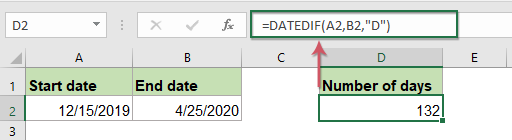Note: In the above formula, A2 indicates the start date, and B2 indicates the end date. You can replace them as your need.

#### Count or Calculate the number of workdays between two dates with formulas

But sometimes, you just want to find out the number of workdays (from Monday to Friday), the above formula will not work for you. In this case, here are two functions can help you to deal with this problem.

##### 1. Using NETWORKDAYS function to count the number of workdays

In a blank cell, please enter the below formula:

=NETWORKDAYS(A2,B2)

Then type Enter key, and you will count the number of workdays excluding Sundays and Saturdays between the two dates. See screenshot: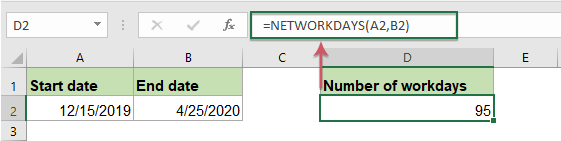Note: In the above formula, A2 indicates the start date, and B2 indicates the end date.

##### 2. Using NETWORKDAYS function to count the number of workdays but exclude the holidays

Sometimes, there may be some holidays during the two date, if you want to calculate the business days between these two dates, you should exclude Saturdays, Sundays, and holidays. This NETWORKDAYS function also can help you.

The syntax for the NETWORKDAYS function is: = NETWORKDAYS ( Start_date , End_date , Holidays )

Type the holiday dates into the cells that you want to subtract from the dates, then enter this formula into a blank cell where you want to get the result:

=NETWORKDAYS(A2,B2,\$C\$2:\$C\$6)

Then press Enter key, the number of workdays which not include Sundays, Saturdays and holidays have been calculated. See screenshot: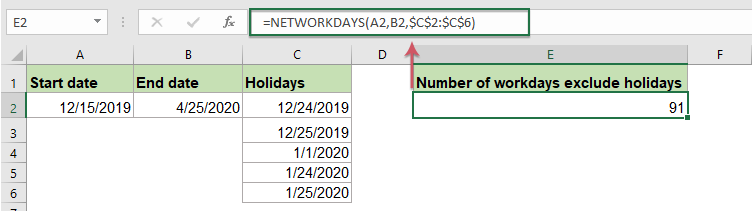Note: In the above formula, A2 indicates start date, B2 stands for end date and C2:C6 is the list of holidays.

##### 3. Using SUM and INT function to count the number of workdays

Except the NETWORKDAYS function, there is another formula could help you to get how many workdays between two dates.

In a blank cell, enter or copy the below formula:

=SUM(INT((WEEKDAY(A2-{2,3,4,5,6})+B2-A2)/7))

And then tap Enter key, and all the workdays will be calculated. See screenshot: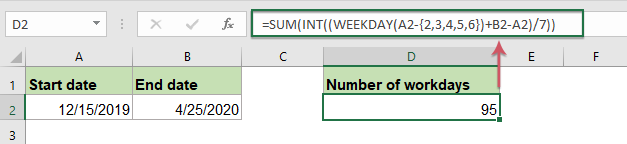Notes:

1. Sometimes, you have 6 workdays per week (from Monday to Saturday), so you need to calculate the number of workdays including Saturdays but excluding Sundays. In this case, you can apply this formula:

=SUM(INT((WEEKDAY(A2-{2,3,4,5,6,7})+B2-A2)/7))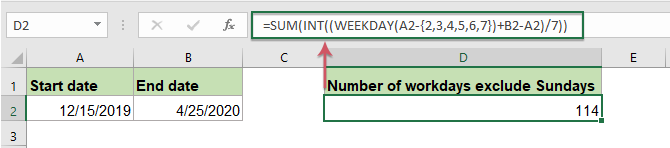2. In the above formulas, A2 stands for the start date and B2 indicates the end date.

#### Count or Calculate the number of weekend days between two dates with formulas

Here also have some formulas to count only the number of weekend days (Saturdays and Sundays) in a given period for you. Please enter or copy any one of the two formulas:

=SUMPRODUCT(--(WEEKDAY(ROW(INDIRECT(A2&":"&B2)),2)>5))
=SUM(INT((WEEKDAY(A2-{1,7})+B2-A2)/7))

Then press Enter key, and now you will get how many weekends between these two dates. See screenshot: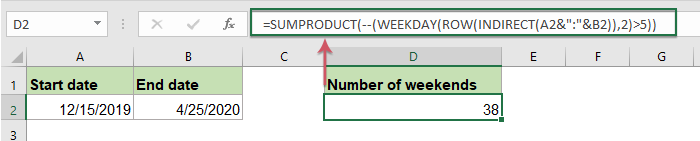Note: In the above formula, A2 indicates start date, B2 stands for end date.

#### Count or Calculate the number of workdays, weekends between two dates with a useful feature

To count the number of weekdays or weekends between two given dates, Kutools for Excel’s Formula Helper utility which contains some commonly used formulas, you can apply them to calculate ages, word appears and so on.

Note:To apply this Formula Helper, firstly, you should download the Kutools for Excel, and then apply the feature quickly and easily.

After installing Kutools for Excel, please do as this:

1. Click a cell where you want to locate the result, and then click KutoolsFormula Helper > Formula Helper, see screenshot: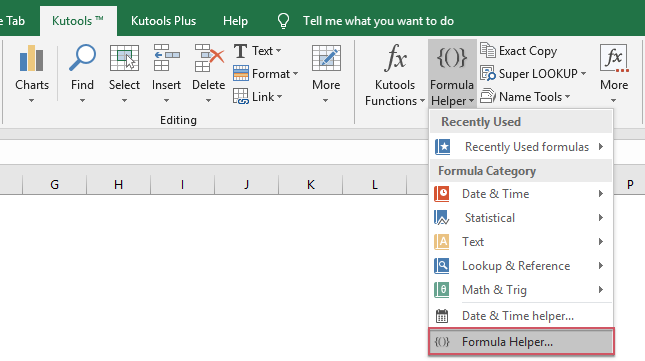2. In the Formulas Helper dialog box, do the following operations:

• Select Statistical option from the Formula Type drop down;
• In the Choose a formula list box, select Number of non-working days between two dates or Number of working days between two dates you want to use;
• Then, in the Arguments input section,select the cells which contain the start date and end date separately.
• At last, click Ok button to get the result as following screesnhot shown: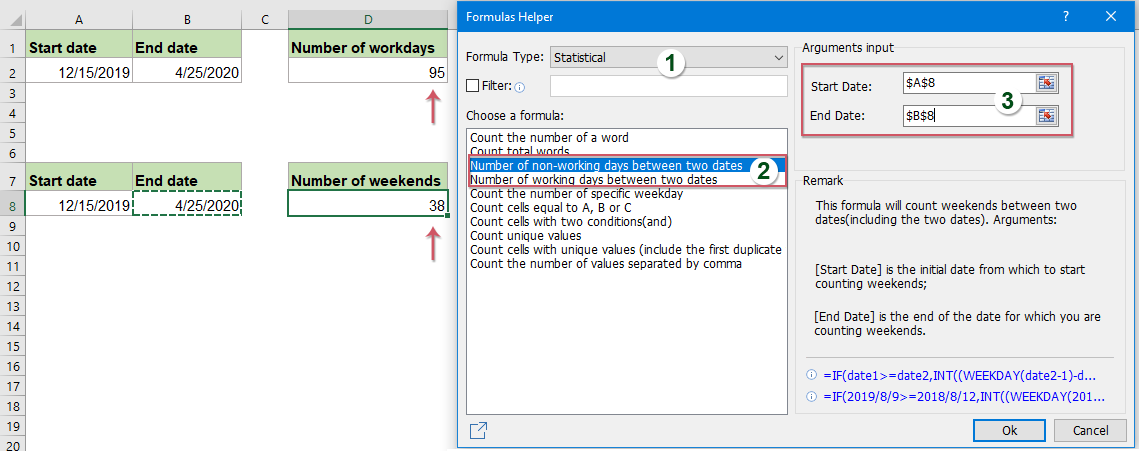#### Count or Calculate the number of days, weeks, months or years between two dates

With Kutools for Excel's Date & Time Helper feature, you can quickly get the various difference results between two dates based on your need without remembering any formulas, such as weeks + days，months + weeks and so on. Click to download Kutools for Excel!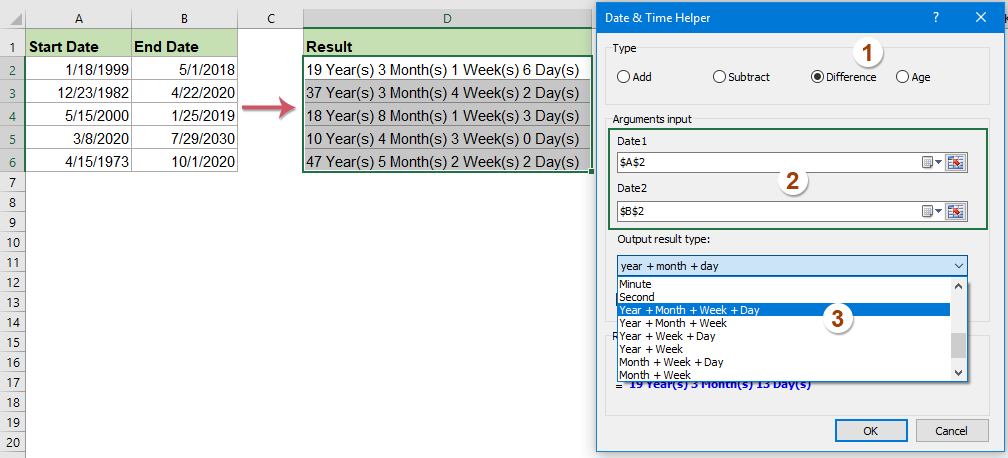Kutools for Excel: with more than 300 handy Excel add-ins, free to try with no limitation in 30 days. Download and free trial Now!

#### More relative date and time articles:

• Calculate Hours Between Times After Midnight In Excel
• Supposing you have a time table to record your work time, the time in Column A is the start time of today and time in Column B is the end time of the following day. Normally, if you calculate the time difference between the two times by directly minus "=B2-A2", it will not display the correct result as left screenshot shown. How could you calculate the hours between two times after midnight in Excel correctly?

### Best Office Productivity Tools

 Popular Features: Find, Highlight or Identify Duplicates   |  Delete Blank Rows   |  Combine Columns or Cells without Losing Data   |   Round without Formula ... Super Lookup: Multiple Criteria VLookup  |   Multiple Value VLookup  |   VLookup Across Multiple Sheets   |   Fuzzy Lookup .... Advanced Drop-down List: Quickly Create Drop Down List   |  Dependent Drop Down List   |  Multi-select Drop Down List .... Column Manager: Add a Specific Number of Columns  |  Move Columns  |  Toggle Visibility Status of Hidden Columns  |  Compare Ranges & Columns ... Featured Features: Grid Focus   |  Design View   |   Big Formula Bar   |  Workbook & Sheet Manager   |  Resource Library (Auto Text)   |  Date Picker   |  Combine Worksheets   |  Encrypt/Decrypt Cells   |  Send Emails by List   |  Super Filter   |   Special Filter (filter bold/italic/strikethrough...) ... Top 15 Toolsets:  12 Text Tools (Add Text, Remove Characters, ...)   |   50+ Chart Types (Gantt Chart, ...)   |   40+ Practical Formulas (Calculate age based on birthday, ...)   |   19 Insertion Tools (Insert QR Code, Insert Picture from Path, ...)   |   12 Conversion Tools (Numbers to Words, Currency Conversion, ...)   |   7 Merge & Split Tools (Advanced Combine Rows, Split Cells, ...)   |   ... and more

Supercharge Your Excel Skills with Kutools for Excel, and Experience Efficiency Like Never Before. Kutools for Excel Offers Over 300 Advanced Features to Boost Productivity and Save Time.  Click Here to Get The Feature You Need The Most...#### Office Tab Brings Tabbed interface to Office, and Make Your Work Much Easier

• Enable tabbed editing and reading in Word, Excel, PowerPoint, Publisher, Access, Visio and Project.
• Open and create multiple documents in new tabs of the same window, rather than in new windows.
• Increases your productivity by 50%, and reduces hundreds of mouse clicks for you every day!No ratings yet. Be the first to rate!
This comment was minimized by the moderator on the site
I am trying to work out how many days worked in a week but I have number of hours worked in the cells. Plus RD as a rest day. is there any way for this to be done rather than manual input
This comment was minimized by the moderator on the site
I am curious if there is a way to have excel do a rolling countdown between dates. Our fiscal year year is 10/1 to 09/30. I figured out how to show how many working days there is between these dates, but now I need to have that number decrease with each passing day. That way each Friday when we open the spreadsheet, it will tell us how many days are left. Is this possible?
This comment was minimized by the moderator on the site
Witam,
chcialabym uzyskać wynik w jednej KOMÓRCE: ZAKRES DAT - czyli np. 08.sie-15.wrz - dane natomiast maja sie zaciagac z innego XLS, w którym zaznaczane jedynkami sa dni urlopu w komórkach poszczeólnych dni. Kolumny w pliku źródłowym to: (scalona komórka MIESIĄCA (sty, lut, mar itp) poniżej są w komórkach wszytskie dni miesiaća 1-31, scalona komorka miesiaca zawiera pod soba wsyztskie te dni
ponizej wiersze dotycza pracowników i w kolejnych kolumnach uzupełniane są JEDNKI - oznaczające wzięcie urlopu w tym dniu....
chciałbym żeby te jedynki były wyciągane z pliku źródłowego tak, aby wynik był zakresem daty....

Czy to jest możliwe?
This comment was minimized by the moderator on the site
Hi,

Can you help me make a formula counting the number of days of work in a week excluding Sundays and holidays, please note Saturday is only a half day of work.

Also I wanted to create a formula to calculate the allowed number of days of leave for employees using the sum of number of year/month/day of service work multiple by 2 working days per month (leave provision per month).

This comment was minimized by the moderator on the site
i have one date, lets say 6/18/2020, i need it has to be minus exact 1 month(5/18/2020), if the result date 5/18/2020 is belongs to saturday or sunday than it would be FRIDAY's date. could you please give the formula for it. - Ramu
This comment was minimized by the moderator on the site
HI,
I NEED TO CALCULATE NUMBER OF DAYS BETWEEN TODAY FROM A STARTING DATE .
This comment was minimized by the moderator on the site
Hi, RAJIH,
You can also use this formula: =DAYS(TODAY(), A2), A2 is the cell contains your starting date, please try, hope it can help you!
Thank you!
This comment was minimized by the moderator on the site
Type your starting date in any cell of excel.
Type today date in another cell of excel.
Now simply minus the starting date from today date.
Example: Starting date is in cell A1, Today date is in cell A2. Simply type =A2-A1.
This comment was minimized by the moderator on the site
This comment was minimized by the moderator on the site
Hello,

To add some days to a date, you just need to add the days to the date directly as below formula:
=A1+60
This comment was minimized by the moderator on the site
HI,
need formula for calculate the days for working days (monday,tuesday, wednesday, thursday and Saturday) - minus holidays. (weekends is friday and sunday)

pls help.
This comment was minimized by the moderator on the site
Offday Friday and Saturday
Public Holiday 22/08/18, 23/08/2018
Manual count for working day are 20 days.
=SUM(INT((WEEKDAY(L3-{1,2,3,4,5})+L5-L3)/7)) --->Total working day 22
=NETWORKDAYS(L14,L16,L18:BS19) -->Total working days 21

This comment was minimized by the moderator on the site
I need formula for 22 working days (Saturday and Sunday OFF)consider in every moth by selecting date range if 21 working days come in a month but considered 22 and if 23 working days come but also considered 22. so please give me any suggestion ?

This comment was minimized by the moderator on the site
I always have Start Date, with set number of working days to complete various activities.

=WORKINGDAY.INTL(START,DAYS,WEEKEND,HOLIDAYS) also causes issues, we work Sun-Thu, so weekend option is 7, yet this formula isn't consistent either, May 2019 has 22 working days (DAYS), this makes the result 02-Jun-19, which is the 2nd working day of June!

Yet same formula for June 2019 with 21 working days gives the expected answer of 30-June-19.
This comment was minimized by the moderator on the site
Hi i need an excel formula which calculates the number of days between two dates and will give me a warning if the count is past day 28. Also if day 28 falls on a weekend or public holiday then the formula needs to account for this so day 28 becomes the next business day.
There are no comments posted here yet